# PWM Generator (Three-phase, Two-level)

Generate three-phase, two-level pulse width modulated waveform

•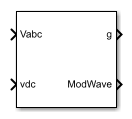Libraries:
Simscape / Electrical / Control / Pulse Width Modulation

## Description

The PWM Generator (Three-phase, Two-level) block controls switching behavior for a three-phase, two-level power converter. The block:

1. Calculates on- and off-gating times based on the block inputs:

• Three sinusoidal reference voltages, one per phase

2. Uses the gating times to generate six switch-controlling pulses.

3. Uses the gating times to generate modulation waveforms.

### Continuous and Discontinuous PWM

The block provides modes for both continuous and discontinuous pulse width modulation (PWM). The figure shows the general difference between continuous sinusoidal PWM (SPWM) and continuous space vector modulation (SVM) waveforms.For discontinuous PWM (DPWM), the block clamps the modulation wave to the positive or negative DC rail for a total of 120 degrees during each fundamental period. During the clamping intervals, modulation discontinues.

A waveform with 30-degree DPWM has four 30-degree intervals per fundamental period.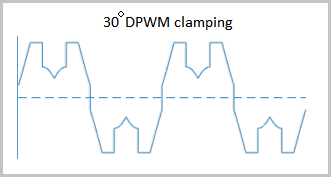Selecting a positive or negative 30-degree phase shift affects the clamping intervals for 60-degree DPWM.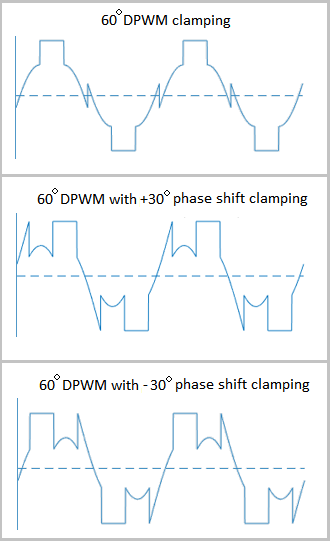The figure shows the waveforms for positive and negative DC clamping for 120-degree DPWM.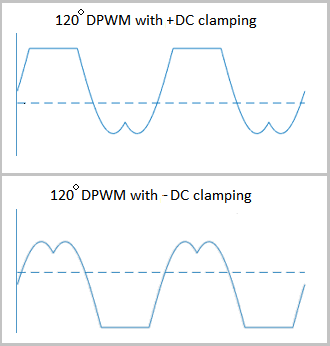### Sampling Mode

This block allows you to choose natural, symmetric, or asymmetric sampling of the modulation wave.

The PWM Generator (Three-phase, Two-level) block does not perform carrier-based PWM. Instead, the block uses input signals to calculate gating times and then uses the gating times to generate both the switch-controlling pulses and the modulation waveforms that it outputs.

Carrier-based PWM is, however, useful for showing how the sampling mode that you select relates to the switch-on and switch-off behavior of the pulses that the block generates. A generator that uses a two-level, carrier-based PWM method:

1. Samples a reference wave.

2. Compares the sample to a triangle carrier wave.

3. Generates a switch-on pulse if a sample is higher than the carrier signal or a switch-off pulse if a sample is lower than the carrier wave.

To determine switch-on and switch-off pulse behavior, a two-level carrier-based PWM generator uses these methods to sample the triangle wave:

• Natural — The sampling and comparison occur at the intersection points of the modulation wave and the carrier wave.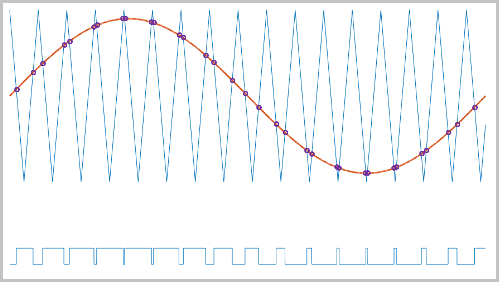• Asymmetric — Sampling occurs at the upper and lower boundaries of the carrier wave. The comparison occurs at the intersection that follows the sampling.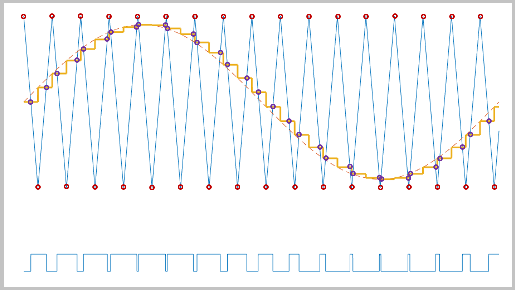• Symmetric — Sampling occurs at only the upper boundary of the carrier wave. The comparison occurs at the intersection that follows the sampling.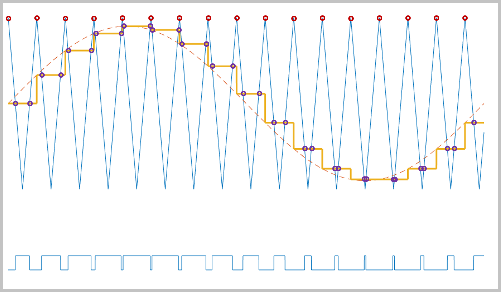### Overmodulation

The modulation index, which measures the ability of the power converter to output a given voltage, is defined as

`$m=\frac{{V}_{M}}{{V}_{C}},$`

where

• m is the modulation index.

• Vm is the peak value of the modulation wave.

• Vc is the peak value of the triangle carrier wave.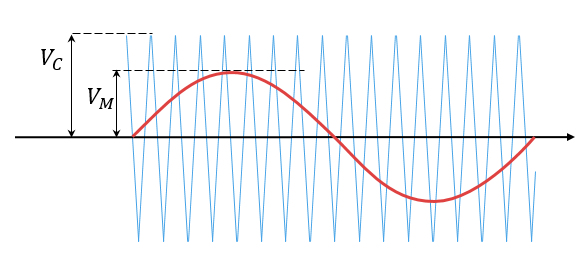For three-phase SPWM,

`${V}_{peak}=m\frac{{v}_{dc}}{2},$`

where

• Vpeak is the peak value of the fundamental component of the phase-to-neutral voltage.

• vdc is the DC-link voltage.

For three-phase space-vector PWM (SVM) and DPWM,

`${V}_{peak}=m\frac{{v}_{dc}}{\sqrt{3}}.$`

For normal steady-state operation, `0` <m`1`. If a transient, such as a load increase, causes the amplitude of Vm to exceed the amplitude of Vc, overmodulation (m > `1`) occurs.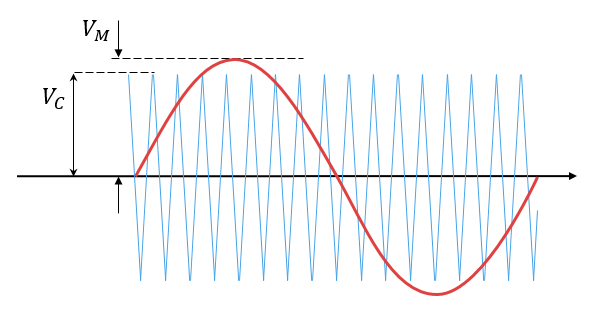If overmodulation occurs, the output voltage of the power converter clamps to the positive or negative DC rail.

In the Three-Phase Two-Level PWM Generator example, the Two-Level Controller subsystem contains a 400–V DC-link input, and a modulation index, m, of 0.8. For SPWM, the maximal input voltage is 400 V/2, that is, 200 V. To demonstrate overmodulation, a transient is added at the beginning of the simulation. The transient forces the amplitudes of the reference voltages to exceed the amplitude of 1/2 of the DC-link voltage. To highlight overmodulation, the scope includes simulation results for only one of the six output pulses and only the a-phase of the reference voltages, modulation waveforms, and output voltages.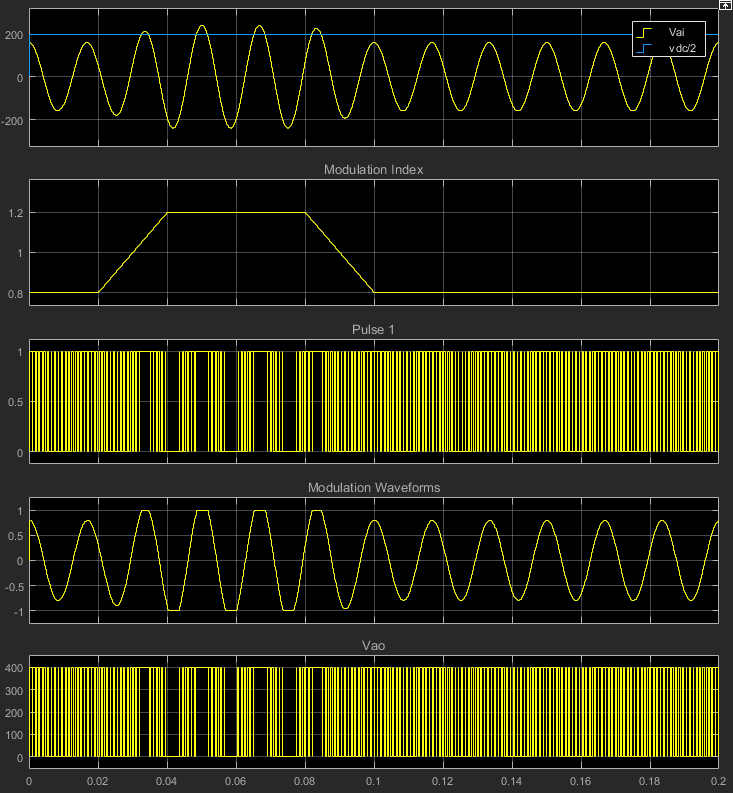The modulation index is greater than one between 0.03–0.09 seconds. During overmodulation:

• The pulse remains in the on or off position.

• The output voltage, Vao, clamps to the positive or negative DC rail.

## Ports

### Input

expand all

Specify the three sinusoidal voltages, one per phase, that you want the attached converter to output.

Specify a positive real number for the DC-link voltage of the converter.

### Output

expand all

Six pulse waveforms that determine switching behavior in the attached power converter.

If you are generating code for a platform that has hardware with PWM capability, you can deploy the modulation wave to the hardware. Otherwise, this data is only for your reference.

## Parameters

expand all

Discontinuous PWM clamps the waveform to the DC rail for a total of 120 degrees in each fundamental period. Continuous PWM does not.

#### Dependencies

The Continuous PWM parameter is only available when you set the PWM mode parameter to `Continuous PWM (CPWM)`.

The sampling mode determines whether the block samples the modulation waveform when the waves intersect or when the carrier wave is at one or both of its boundary conditions.

Specify the rate at which you want the switches in the power converter to switch.

Specify the time interval between successive block executions (output calculations). To ensure adequate resolution in the generated signal, set this value to be less than or equal to `1/(50*Fsw)`, where Fsw is the Switching frequency (Hz).

Specify the method for distributing the 120 degrees per period during which the block clamps the modulation wave to the DC rail. Other options are:

• ```60 DPWM (+30 degree shift): +30 degree shift from 60 DPWM```

• ```60 DPWM (-30 degree shift): -30 degree shift from 60 DPWM```

• ```30 DPWM: 30 degree discontinuous PWM```

• ```120 DPWM: positive dc component```

• ```120 DPWM: negative dc component```

When the wave is clamped, modulation discontinues.

#### Dependencies

The Discontinuous PWM parameter is only available when you set the PWM mode parameter to `Discontinuous PWM (DPWM)`.

 Chung, D. W., J. S. Kim, and S. K. Sul. “Unified Voltage Modulation Technique for Real Time Three-Phase Power Conversion.” IEEE Transactions on Industry Applications, Vol. 34, No. 2, 1998, pp. 374–380.

 Hava, A. M., R. J. Kerkman, and T. A. Lipo. “Simple Analytical and Graphical Methods for Carrier-Based PWM-VSI Drives.” IEEE Transactions on Power Electronics, Vol. 14, No. 1, 1999, pp. 49–61.